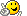## Recommended Posts

Hi all,

i want to create an array that contains pixel colours. I want to scan a client area for all the colors it contains and enter all the colors  found(excluding duplicates) in an array called  \$get_pix.color.So how can i do that??

Edited by NileshSutar

##### Share on other sites

You cannot have a "." in a variable name.

They are used as operators in objects.

Show what code you have.

Monkey's are, like, natures humans.

##### Share on other sites

You cannot have a "." in a variable name.

They are used as operators in objects.

Show what code you have

The code is :

```Func start_capture()
For \$i=\$x1 To \$x2 Step 1
For \$j=\$y1 To \$y2 Step 1
For \$s=0 To <??> Step 1; Don't know how many pixels will be  there. What to do?
\$pixel[\$s] = PixelGetColor(\$i,\$j,2)
Next
Next
Next
EndFunc```

##### Share on other sites

The area tou are working on should give you the size of the array you need.

If the area width is 100 and height is 100, then you need 10000 elements.

Monkey's are, like, natures humans.

##### Share on other sites

The code is :

` `

now i have modified it:

```global \$Area=(\$x2-\$x1)*(\$y2-\$y1)
global \$x1
global \$x2
global \$y1
global \$y2
Func start_capture()
For \$i=\$x1 To \$x2 Step 1
For \$j=\$y1 To \$y2 Step 1
For \$s=0 To \$Area Step 1; Don't know how many pixels will be  there. What to do?
\$pixel[\$s] = PixelGetColor(\$i,\$j,2)
Next
Next
Next
EndFunc```

##### Share on other sites

Why not use a 2D array, for example...

```#include <Array.au3>

\$Width = 10
\$Height = 10
\$StartX = 0
\$StartY = 0

Local \$aPixels[\$Height][\$Width]

For \$y = \$StartY To \$Height -1
For \$x = \$StartX To \$Width -1
\$aPixels[\$y][\$x] = "0x" & Hex(PixelGetColor(\$x,\$y),6)
Next
Next

_ArrayDisplay(\$aPixels) ```

To make it more generic you need to apply a little maths logic.

Edited by JohnOne

Monkey's are, like, natures humans.

##### Share on other sites

Why not use a 2D array, for example...

```#include <Array.au3>

\$Width = 10
\$Height = 10
\$StartX = 0
\$StartY = 0

Local \$aPixels[\$Height][\$Width]

For \$y = \$StartY To \$Height -1
For \$x = \$StartX To \$Width -1
\$aPixels[\$y][\$x] = "0x" & Hex(PixelGetColor(\$x,\$y),6)
Next
Next

_ArrayDisplay(\$aPixels) ```

To make it more generic you need to apply a little maths logic.

i tested the code.I found that the coordinates \$StartX and  \$StartY must be zero for the script to run.Otherwise it will not five results.I figured out why this is happening but i am unable to change the code.So the \$y and \$x values are not zero when i set \$StartX and \$StartY to some non-zero.So as they are input to the pixel array the array is getting confused.We should some how set \$y=0 and increament its value without affecting PixelGetColor(\$x,\$y).

##### Share on other sites

Like I said, a little maths logic is all it takes.

The start x and y can be whatever you want so long as you as add to it the width and height.

Monkey's are, like, natures humans.

##### Share on other sites

The start x and y can be whatever you want so long as you as add to it the width and height.

```For \$y = \$StartY To \$Height -1
For \$x = \$StartX To \$Width -1
For \$i=0 To (\$Width-\$StartX+1)*(\$Height-\$StartY+1)  +1
\$aPixels[\$i] = ("0x" & Hex(PixelGetColor(\$x,\$y))
Next
Next

Next```

I have made it like that but it is giving me an error "Array variable has incorrect number of subscripts or subscript dimension range exceeded"

##### Share on other sites

Look at the for loop, if start y is 300 and height is 20, the loop will be going from 300 to 20.

Hint

For \$y = \$StartY To ? + \$Height -1

EDIT:

Apply reverse logic to your array.

Edited by JohnOne

Monkey's are, like, natures humans.

##### Share on other sites

Look at the for loop, if start y is 300 and height is 20, the loop will be going from 300 to 20.

Hint

For \$y = \$StartY To ? + \$Height -1

EDIT:

Apply reverse logic to your array.

`For \$y = \$StartY To \$StartY + \$Height -1;is it right`
Edited by NileshSutar

##### Share on other sites

John i think that the thing that is going wrong is the

`\$aPixels[\$y][\$x] = "0x" & Hex(PixelGetColor(\$x,\$y),6)`

When \$y and \$x are zero then there is no problem for \$aPixels to take values in.But when \$y and \$x are non-zero the first element   should get 0 index(in array \$aPixels) will be told to take \$y index which is not zero. I think this is a big problem.

Edited by NileshSutar

##### Share on other sites

Off top of my head it'd be something like this

```#include <Array.au3>

\$Width = 10
\$Height = 10
\$StartX = 0
\$StartY = 0

Local \$aPixels[\$Height][\$Width]

For \$y = \$StartY To \$StartY + \$Height -1
For \$x = \$StartX To \$StartX + \$Width -1
\$aPixels[\$y - \$StartY][\$x - \$StartX] = "0x" & Hex(PixelGetColor(\$x,\$y),6)
Next
Next

_ArrayDisplay(\$aPixels)```

Monkey's are, like, natures humans.

##### Share on other sites

Off top of my head it'd be something like this

```#include <Array.au3>

\$Width = 10
\$Height = 10
\$StartX = 0
\$StartY = 0

Local \$aPixels[\$Height][\$Width]

For \$y = \$StartY To \$StartY + \$Height -1
For \$x = \$StartX To \$StartX + \$Width -1
\$aPixels[\$y - \$StartY][\$x - \$StartX] = "0x" & Hex(PixelGetColor(\$x,\$y),6)
Next
Next

_ArrayDisplay(\$aPixels)```

lol working.##### Share on other sites

Ace.

Just pop in a function now and use those non scoped vars as parameters.

Monkey's are, like, natures humans.

## Create an account

Register a new account

• ### Recently Browsing   0 members

×

• Wiki

• Back

• #### Beta

• Git
• FAQ
• Our Picks
×
• Create New...